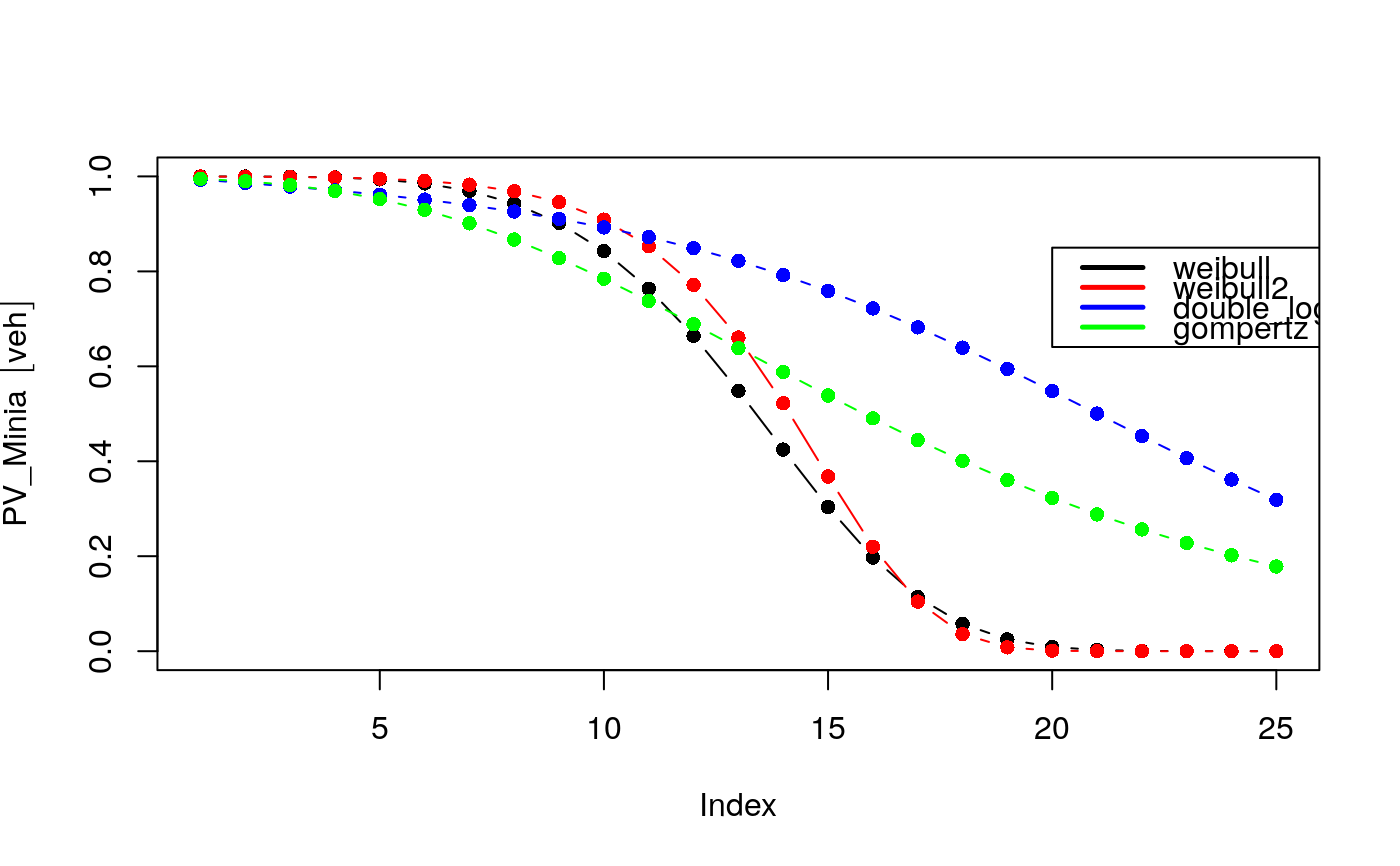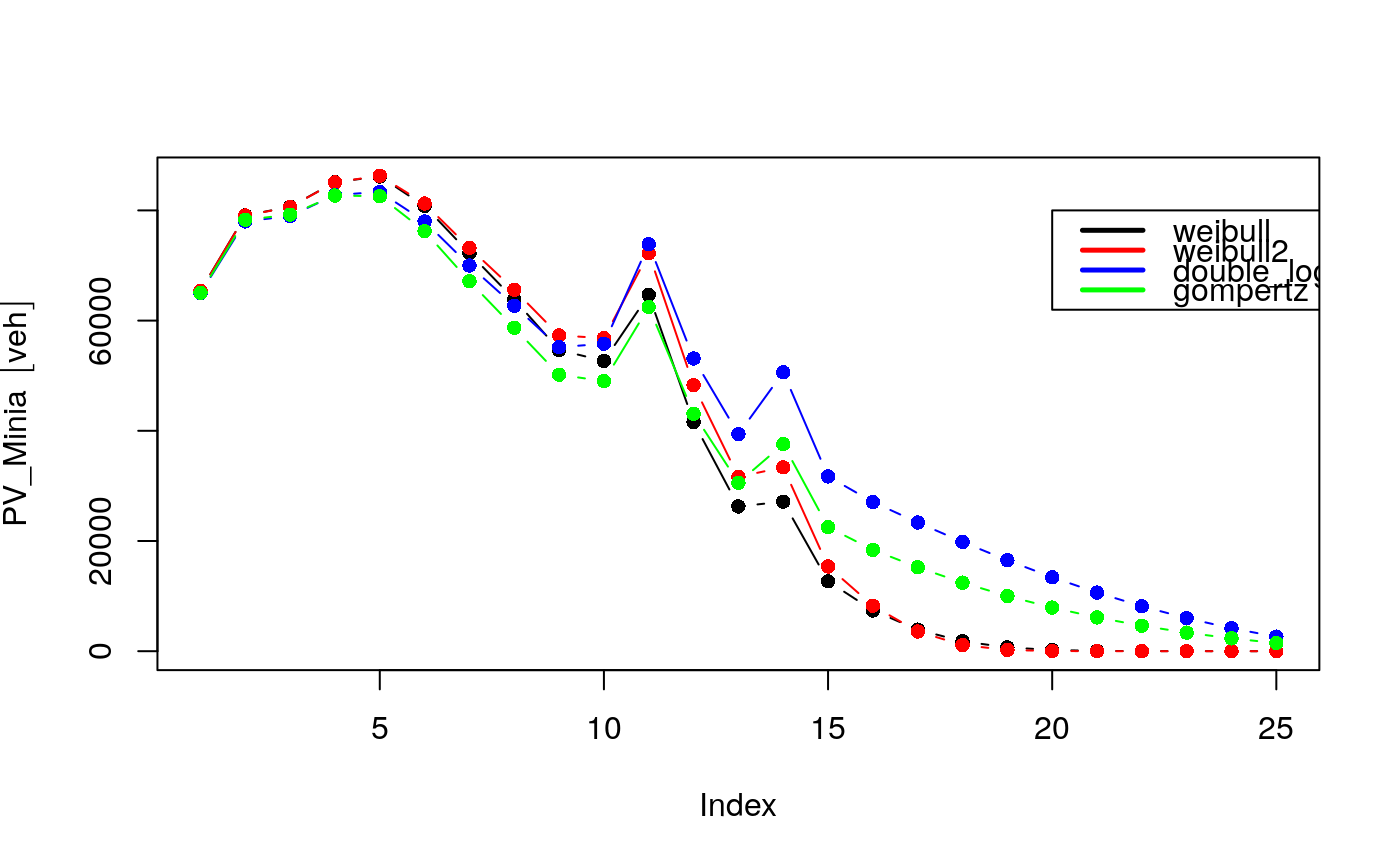age returns survived vehicles

age(x, type = "weibull", a = 14.46, b = 4.79, agemax, net,
verbose = FALSE)

Arguments

x Numeric; numerical vector of sales or registrations for each year Character; any of "gompertz", "double_logistic", "weibull" and "weibull2" Numeric; parameter of survival equation Numeric; parameter of survival equation Integer; age of oldest vehicles for that category SpatialLinesDataFrame or Spatial Feature of "LINESTRING" Logical; message with average age and total numer of vehicles regions or streets.

Value

dataframe of age distrubution of vehicles

Note

The functions age* produce distribution of the circulating fleet by age of use. The order of using these functions is:

1. If you know the distribution of the vehicles by age of use , use: my_age 2. If you know the sales of vehicles, or the registry of new vehicles, use age to apply a survival function. 3. If you know the theoretical shape of the circulating fleet and you can use age_ldv, age_hdv or age_moto. For instance, you dont know the sales or registry of vehicles, but somehow you know the shape of this curve. 4. You can use/merge/transform/dapt any of these functions.

gompertz: 1 - exp(-exp(a + b*time)), defaults PC: b = -0.137, a = 1.798, LCV: b = -0.141, a = 1.618 MCT (2006). de Gases de Efeito Estufa-Emissoes de Gases de Efeito Estufa por Fontes Moveis, no Setor Energético. Ministerio da Ciencia e Tecnologia. This curve is also used by Guo and Wang (2012, 2015) in the form: V*exp(alpha*exp(beta*E)) where V is the saturation car ownership level and E GDP per capita Huo, H., & Wang, M. (2012). Modeling future vehicle sales and stock in China. Energy Policy, 43, 17–29. doi:10.1016/j.enpol.2011.09.063 Huo, Hong, et al. "Vehicular air pollutant emissions in China: evaluation of past control policies and future perspectives." Mitigation and Adaptation Strategies for Global Change 20.5 (2015): 719-733.

double_logistic: 1/(1 + exp(a*(time + b))) + 1/(1 + exp(a*(time - b))), defaults PC: b = 21, a = 0.19, LCV: b = 15.3, a = 0.17, HGV: b = 17, a = 0.1, BUS: b = 19.1, a = 0.16 MCT (2006). de Gases de Efeito Estufa-Emissoes de Gases de Efeito Estufa por Fontes Moveis, no Setor Energético. Ministerio da Ciencia e Tecnologia.

weibull: exp(-(time/a)^b), defaults PC: b = 4.79, a = 14.46, Taxi: b = +inf, a = 5, Government and business: b = 5.33, a = 13.11 Non-operating vehicles: b = 5.08, a = 11.53 Bus: b = +inf, a = 9, non-transit bus: b = +inf, a = 5.5 Heavy HGV: b = 5.58, a = 12.8, Medium HGV: b = 5.58, a = 10.09, Light HGV: b = 5.58, a = 8.02 Hao, H., Wang, H., Ouyang, M., & Cheng, F. (2011). Vehicle survival patterns in China. Science China Technological Sciences, 54(3), 625-629.

weibull2: exp(-((time + b)/a)^b ), defaults b = 11, a = 26 Zachariadis, T., Samaras, Z., Zierock, K. H. (1995). Dynamic modeling of vehicle populations: an engineering approach for emissions calculations. Technological Forecasting and Social Change, 50(2), 135-149. Cited by Huo and Wang (2012)

Examples

{ vehLIA <- rep(1, 25) PV_Minia <- age(x = vehLIA) PV_Minib <- age(x = vehLIA, type = "weibull2", b = 11, a = 26) PV_Minic <- age(x = vehLIA, type = "double_logistic", b = 21, a = 0.19) PV_Minid <- age(x = vehLIA, type = "gompertz", b = -0.137, a = 1.798) plot(PV_Minia, type = "b", pch = 16) lines(PV_Minib, type = "b", pch = 16, col = "red") lines(PV_Minic, type = "b", pch = 16, col = "blue") lines(PV_Minid, type = "b", pch = 16, col = "green") legend(x = 20, y = 0.85, legend = c("weibull", "weibull2", "double_logistic", "gompertz"), col = c("black", "red", "blue", "green"), lty=c(1,1), lwd=c(2.5, 2.5, 2.5, 2.5)) #lets put some numbers vehLIA <- c(65400, 79100, 80700, 85300, 86700, 82000, 74500, 67700, 60600, 62500, 84700, 62600, 47900, 63900, 41800, 37492, 34243, 30995, 27747, 24499, 21250, 18002, 14754, 11506, 8257) PV_Minia <- age(x = vehLIA) PV_Minib <- age(x = vehLIA, type = "weibull2", b = 11, a = 26) PV_Minic <- age(x = vehLIA, type = "double_logistic", b = 21, a = 0.19) PV_Minid <- age(x = vehLIA, type = "gompertz", b = -0.137, a = 1.798) plot(PV_Minia, type = "b", pch = 16) lines(PV_Minib, type = "b", pch = 16, col = "red") lines(PV_Minic, type = "b", pch = 16, col = "blue") lines(PV_Minid, type = "b", pch = 16, col = "green") legend(x = 20, y = 80000, legend = c("weibull", "weibull2", "double_logistic", "gompertz"), col = c("black", "red", "blue", "green"), lty=c(1,1), lwd=c(2.5, 2.5, 2.5, 2.5)) }